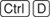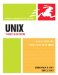# Calculating with bc

### Calculating with bc

Unix even offers a handy calculator utility that lets you...er, um...calculate things. Just use bc, as shown in Code Listing 15.2.

##### Code Listing 15.2. Using the bc utility, you can calculate and calculate and calculate...

 ` xmission> bc 6*5 30 xmission> `

#### To Calculate with bc:

 1 bc At the shell prompt, type bc. You'll find yourself at a blank line, waiting for math to do. 2 6*5 Enter the numbers, operators, expressions, or whatever you want to calculate. Use + to add, - to subtract, * to multiply, and / to divide. The answer appears on the next line (Code Listing 15.2). 3Quit bc when you're done.Tips

• You can tell bc to calculate expressions within a file with bc filename.(Of course, replace filename with the real filename.) Then, bc waits for more to do from the command line.

• Type man bc for more details about bc's capabilities.UNIX, Third Edition
ISBN: 0321442458
EAN: 2147483647
Year: 2006
Pages: 251

Similar book on Amazon